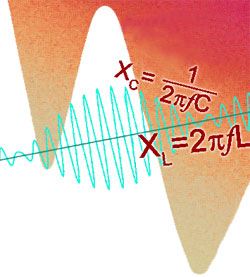# Reactance

• Section 6.1 Inductive Reactance.
• •Inductive Reactance.
• •The relationship between reactance, frequency and inductance.
• •Graphical representation of inductive reactance.
• Section 6.2 Capacitive Reactance.
• •Capacitive Reactance.
• •The relationship between reactance, frequency and capacitance.
• •Graphical representation of capacitive reactance.
• Section 6.3 XL Calculations.
• • Calculations involving inductive reactance.
• • Multi stage calculations.
• Section 6.4 XC Calculations.
• • Calculations involving capacitive reactance.
• • Multi stage calculations involving reactance & resistance.
• Section 6.5 Reactance Quiz.
• • Choosing standard formulae to solve inductive and capacitive reactance problems.
• • Using Multi stage calculations involving inductive and capacitive reactance & resistance.

## Reactance and ResistanceResistance is unaffected by the rate of change of voltage or current and remains the same whether DC, or AC of any frequency is applied. In a purely resistive circuit Ohms law applies and V = IR. Calculations of resistance in AC circuits are the same as for DC. The only extra thing to remember when calculating AC values, is that the same type of value, e.g. the RMS, Average value, or the peak-to-peak value must be used for all AC voltages or currents throughout the equation. Inductance and capacitance however, have an effect on current which depends on frequency, and when either component is used with AC voltages and currents, then the frequency of the waveform must be taken into account.

Inductive and capacitive reactance have opposite effects in relation to frequency. Inductive reactance rises with an increase in frequency, and capacitive reactance falls. Both are similar to resistance and are measured in Ohms, but resistance remains the same, whatever the frequency. The interplay between resistance and reactance will form the basis of many effects in the later modules.